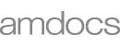# Placement Papers - AmdocsAMDOCS PAPER - 06 JUL 2007
Posted by :
S.tamilarasan
(56)
Hi Friends

Amdocs came to our campus for placements on July 6th, 2007. This year they have changed the pattern. It was only 2 hours exam. They have removed the pseudo code section. Total 7 sections were there - 4 for aptitude and 3 for technical (C / C++ / JAVA, SQL, Unix). They have selected all the candidates who opted for JAVA in the technical section.

There were 95 questions in total. There was sectional cut off of 60% for aptitude section. For technical section, there was no sectional cut off. But in total as usual 60% cut off was there. The total paper was objective. 0.25 negative marking was there.

Section: I
1. Analytical Ability - 20q
2. Pattern Matching - 15q
3. Comprehension - 10q

Section: II
1. C / C++ / Java - We can choose one of them - 20q
2. SQL - 15q
3. Unix - 15q

Analytical Ability
I remember only very few questions. First two questions related to sets. In a forest in total 42 animals are there of which 21 have 4 legs n 30 have horns n 5 have both. Then
1. How many animals are there with only 4 legs
2. How many animals are there with only horns

3. There is a die. We can see only three sides of the die at a time. When 3 was on the top 2, 4 were on the adjacent sides like that 2 more cases were given and we have to find out the number which is on the opposite side of 2

Questions 5-10 are alike. Two statements n one question will be there. All of them will be having the same options of
a. The question can be answered with help of only Statement I.
b. With help of only Statement II.
c. We need both the statements to answer the questions
d. We can’t answer the question at all.

And I remember some questions among them

What is the value of sin ½ ? n the statements are
a. sin½cos½=0
b. sin½-cos½=0

What is the value of n?
a. k3-n3=0
b. k=0

Is A=1?
a. 3A2/5A2=3/5
b. AB=1
…..like that

Questions 12-16 will be having some number conversions. In general they will give the binary to decimal conversion indirectly. Like for us they have given the following instructions.

In a number system, \$ represents 1 and * represents 0. \$ when shifted to left, will be multiplied by 2. So 4 is represented as \$**.
And they have given the questions like evaluate the expression like that. And in another paper I saw that they have given the same pattern except that @ represents 1 and # represents 0.

Pattern Matching
In pattern Matching the following options were there for questions 1-3.
A. The expression is same as A&C
B. The expression is same as B&C
C. The expression is same as A&B
D. All are same.
E. None of them are same.

Sample question:
1. nnMMM@7.34
A. nnMMM@7.34 B.nnMMM@734 C. nnMMM@7.34

Be careful in this section
2. In some system + represents *, - represents +, * represents / and / represents- then evaluate the following expression 6/8-3*6+4
3. Similar kind of question with different system

For Questions 6-10 they have given selection criteria of a company and we have to find out whether the given candidate is eligible or not like

The candidate need the following:
1. He should have 60% till 12th in all the examinations
2. He should have minimum of 65% in graduation and 70% in post graduation

And he should satisfy atleast one of the following conditions:
1. He should have recommendation letter.
2. He should be an athlet in atleast state level

1. Rahul have 62% in 12th, 75% in graduation n 80% in PG. He was tennis champion in university Level. (Ans: not eligible)

Questions 11-15 also have the same type of questions but the selection criteria is different.

Comprehension
We were given a comprehension related to sequential, direct memory addressing, indexing n hashing and based on that 10q were there.

C

1. What is the output?
Struct ee
{
int i;}
Main()
{
Printf(“main()”);
} (Note: Semicolon is missing after struct)

2.What is the output?
Main()
{
Int I=5;
For(i=5;i>=0;i--)
{
Printf(“%d”,i); Main();
}
} (Note:I is not a static variable)

3. What is the output?
Main()
{
Char *p;
p=fun();
Printf(“%s”,*p);
}
Char* fun()
{
Char b[]=”hello”;
Return b;
}

Some more questions on strings, One question was there on enumerations…. Like that…..

SQL
Most of the questions u can answer only if u know PL/SQL n with some knowledge of ORACLE.

UNIX
My seniors told me that in previous papers shell scripting was not there but this year we have some questions on shell scripting too. So be prepared for that.
1. What is the output? - shell scripting for loop and command line arguments
2. One question on \$\$ - used for shell variables
3. What happens when we give cal j 1997—j is for jan, june, july
4. One question on pipes using tee